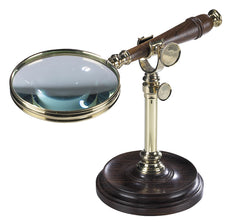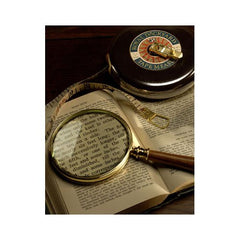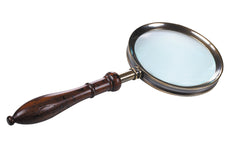# History of Magnifying Glasses

The earliest evidence of a magnifying device was a joke in Aristophanes's The Clouds from 424 BC, where magnifying lenses to start kindling were sold in a pharmacy, and Pliny the Elder's "lens", a glass globe filled with water, used to cauterize wounds. (Seneca wrote that it could be used to read letters "no matter how small or dim"). Roger Bacon described the properties of a magnifying glass in 13th-century England. Eyeglasses were developed in 13th-century Italy.The magnification of a magnifying glass depends upon where it is placed between the user's eye and the object being viewed, and the total distance between them. The magnifying power is equivalent to angular magnification (this should not be confused with optical power, which is a different quantity). The magnifying power is the ratio of the sizes of the images formed on the user's retina with and without the lens. For the "without" case, it is typically assumed that the user would bring the object as close to one eye as possible without it becoming blurry. This point, known as the near point, varies with age. In a young child, it can be as close as 5 cm, while, in an elderly person it may be as far as one or two metres. Magnifiers are typically characterized using a "standard" value of 0.25 m.The highest magnifying power is obtained by putting the lens very close to one eye and moving the eye and the lens together to obtain the best focus. The object will then typically also be close to the lens. The magnifying power obtained in this condition is MP0 = (0.25 m)Φ + 1, where Φ is the optical power in dioptres, and the factor of 0.25 m represents the assumed near point (¼ m from the eye). This value of the magnifying power is the one normally used to characterize magnifiers. It is typically denoted "m×", where m = MP0. This is sometimes called the total power of the magnifier (again, not to be confused with optical power).

However, magnifiers are not always used as described above because it is more comfortable to put the magnifier close to the object (one focal length away). The eye can then be a larger distance away, and a good image can be obtained very easily; the focus is not very sensitive to the eye's exact position. The magnifying power in this case is roughly MP = (0.25 m)Φ.A typical magnifying glass might have a focal length of 25 cm, corresponding to an optical power of 4 dioptres. Such a magnifier would be sold as a "2×" magnifier. In actual use, an observer with "typical" eyes would obtain a magnifying power between 1 and 2, depending on where lens is held.